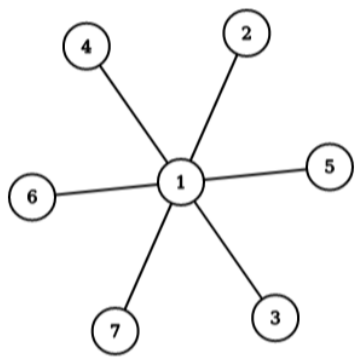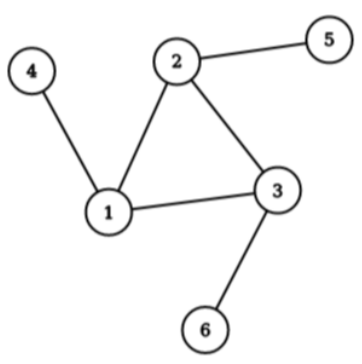시간 제한메모리 제한제출정답맞은 사람정답 비율
2 초 1024 MB0000.000%

## 문제

Ulovi me, ulovi me, kupit ću ti novine!” – a popular play song among Croatian children. Translates to catch me, and I’ll buy you newspapers.

Ankica and Branko are playing a chasing game on an undirected, connected graph. Namely, Branko is moving around the graph, while Ankica is attempting to catch him. The game proceeds in turns, and a single turn consists of the following:

• Ankica makes a guess on Branko’s whereabouts. More precisely, she guesses that Branko is currently located at a specific node. If she guesses correctly, Branko is caught and the game ends. Otherwise,
• Branko traverses an edge incident to his current location. In other words, Branko moves to one of his neighbouring nodes. Note that Branko cannot stay at his present location.

Given a graph, determine if Ankica has a finite strategy which always catches Branko regardless of the way Branko plays and what his starting position may be.

More formally, we represent Ankica’s strategy as an array $A = (a_1, a_2, \dots , a_k)$, where $a_i$ denotes Ankica’s guess in the $i$-th turn (i.e. she guesses that Branko is located in the node $a_i$).

Similarly, we represent Branko’s movements as an array $B = (b_1, b_2, \dots , b_k)$, where $b_i$ represents the node in which Branko is located before the $i$-th turn. Additionally, for each two successive elements $b_i$ and $b_{i+1}$ ($1 \le i < k$), there must exist an edge in the graph connecting nodes $b_i$ and $b_{i+1}$. Note that no such constraint is imposed on array $A$.

We say that Ankica’s strategy is successful, i.e. she catches Branko in at most $k$ turns, if, for every valid array $B$ of length $k$, there exists some $i$ ($1 \le i \le k$) such that $a_i = b_i$ holds.

If such strategy exists, you should find one that minimizes the number $k$.

You can score some points in this task if you are able to provide a succesful, but not optimal, strategy for Ankica (i.e. a strategy where $k$ is not minimal). See the Scoring section for more details.

## 입력

The first line contains two integers $N$ and $M$ ($N - 1 \le M \le \frac{N(N-1)}{2}$) that represent the number of nodes and edges in the graph (respectively). Nodes of the graph are denoted with integers from 1 to $N$.

The $i$-th of the next $M$ lines contains two space-separated integers $u_i$ and $v_i$ ($1 \le u_i, v_i \le N$, $u_i \ne v_i$), representing that an undirected edge connects nodes $u_i$ and $v_i$. No edge will appear more than once in the input, and the graph will be connected.

## 출력

If there is no successful strategy for Ankica, simply output "NO" in the first line and terminate the program.

Otherwise, you should output "YES" in the first line.

The second line should contain the number $k$ from the task description. The third line should contain $k$ numbers $a_1, a_2, \dots , a_k$ from the task description.

## 서브태스크

번호 배점 제한
1 12

$1 \le N \le 20$

2 8

$1 \le N \le 1\,000$, $M = N - 1$, each node $u = 1, \dots , N - 1$ is connected to node $u + 1$

3 80

$1 \le N \le 1\,000$

If your program correctly outputs "YES" on the first line on a specific test case, but fails to provide a successful strategy, that test case will be scored with 50% of the points allocated for the subtask it is a part of.

If your program correctly outputs "YES" on the first line and provides a successful, but not optimal strategy, that test case will be scored with 75% of the points allocated for the subtask it belongs to. Additionally, in order to score these points, the number of turns ($k$) in the outputted strategy must be at most $5N$. It can be proved that the number of turns in the optimal strategy doesn’t exceed $5N$.

The score for each subtask equals to the smallest score obtained by one of its test cases.

## 예제 입력 1

7 6
1 2
1 3
1 4
1 5
1 6
1 7


## 예제 출력 1

YES
2
1 1If Branko is initially located at node 1, he will be caught on the first turn. Otherwise, he will be caught on the second turn.

## 예제 입력 2

6 6
1 2
2 3
3 1
1 4
2 5
3 6


## 예제 출력 2

NOLet Branko’s initial location be among nodes 1, 2 or 3, and different from $a_1$. Each of these nodes is connected to the other two, so Branko has two options to move to during each turn. At least one option must be safe, so Ankica has no successful strategy.

## 채점 및 기타 정보

• 예제는 채점하지 않는다.